# RD Sharma Solutions for Class 8 Maths Chapter 3 - Squares and Square Roots Exercise 3.1

The pdf of RD Sharma Solutions for the Exercise 3.1 of Class 8 Maths Chapter 3, Squares and Square Roots are available here. The questions present in Exercise 3.1 have been solved by BYJU’S experts in Maths, and this will help students in solving the questions without any error. As this is the first exercise, this will help the students to understand the basic concepts of the perfect square as well as the prime factorisation method.

By practising the RD Sharma Solutions for Class 8, students will be able to grasp the concepts perfectly. Hence, students whose aim is to score high in Maths examinations are advised to go through RD Sharma Class 8 Solutions textbook.

## Download the pdf of RD Sharma Solutions For Class 8 Maths Chapter 3 Squares and Square Roots Exercise 3.1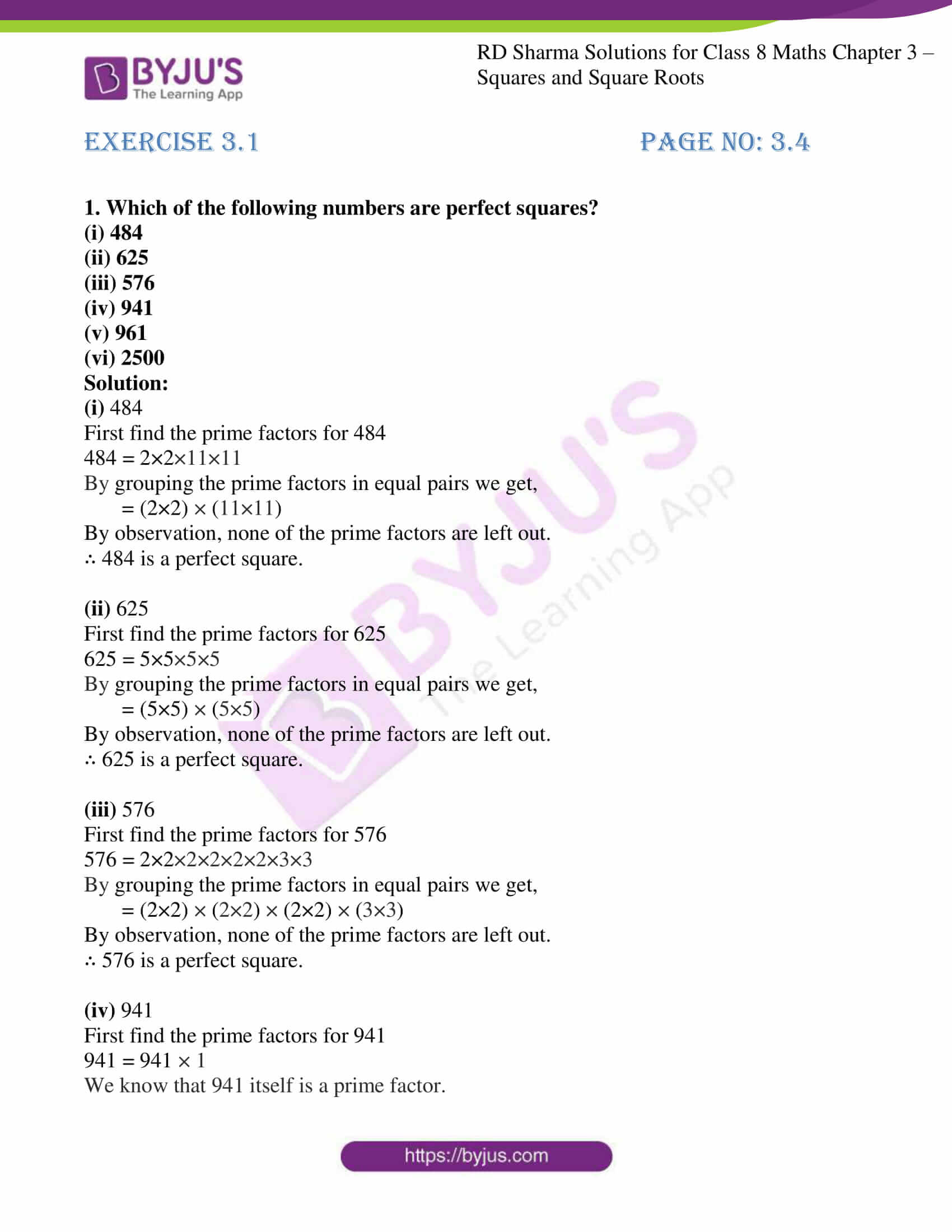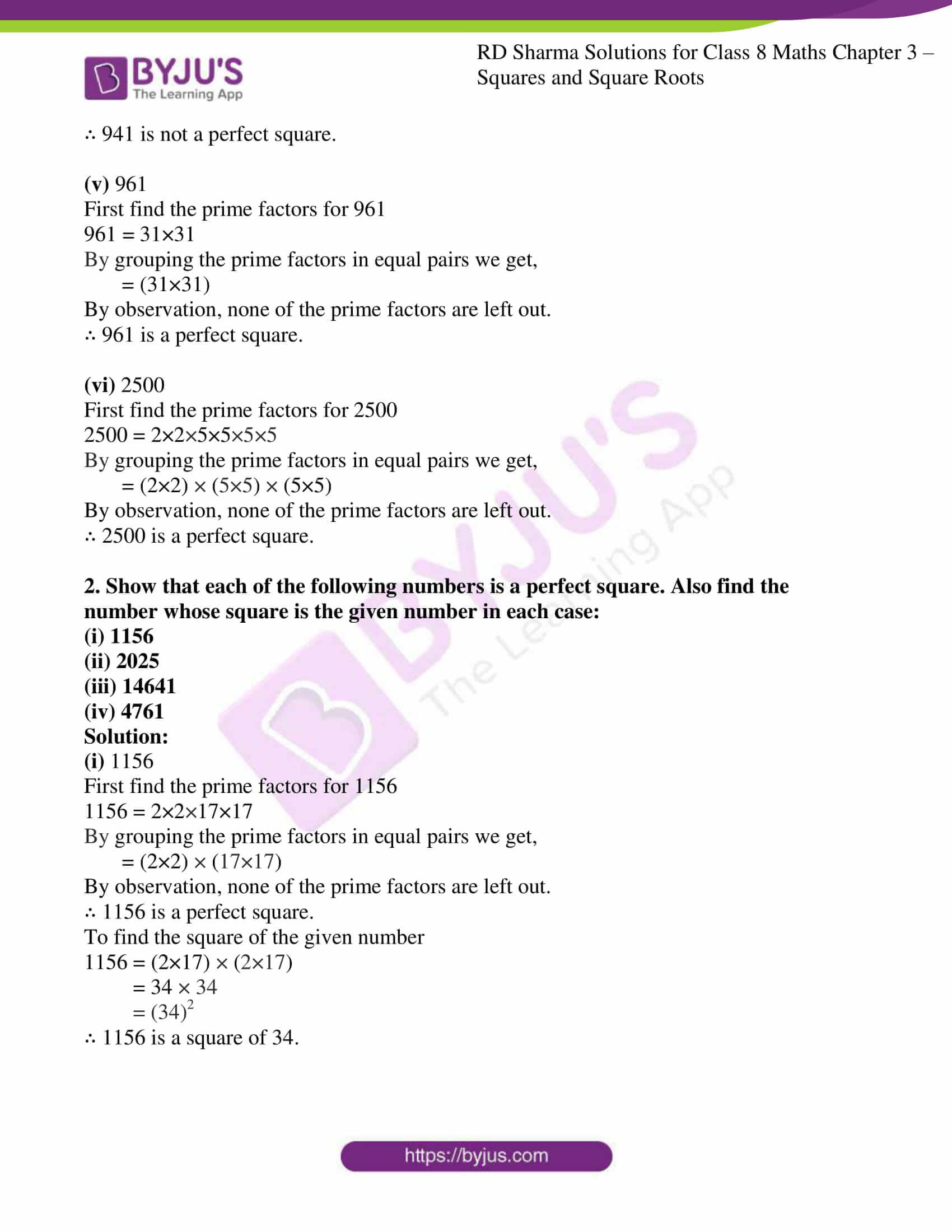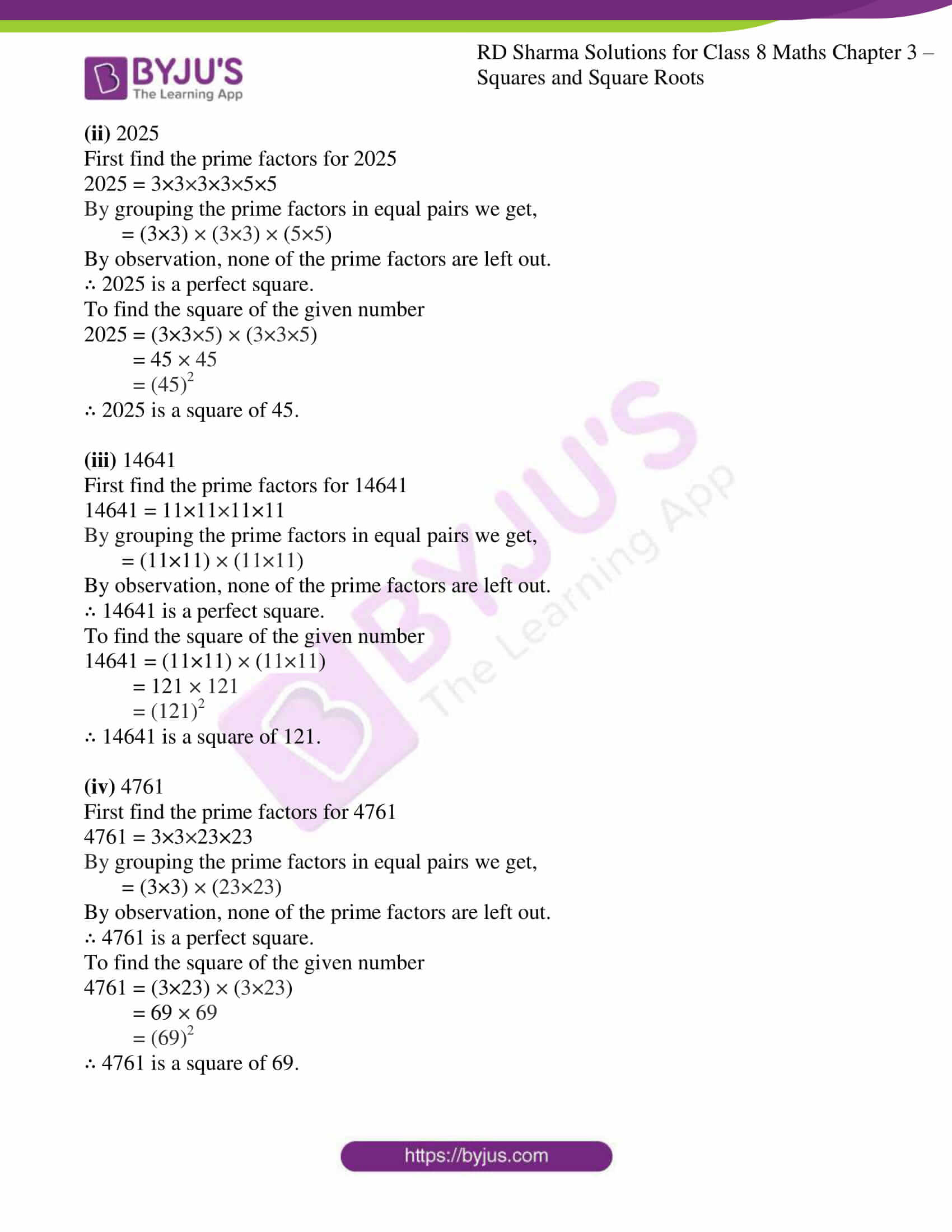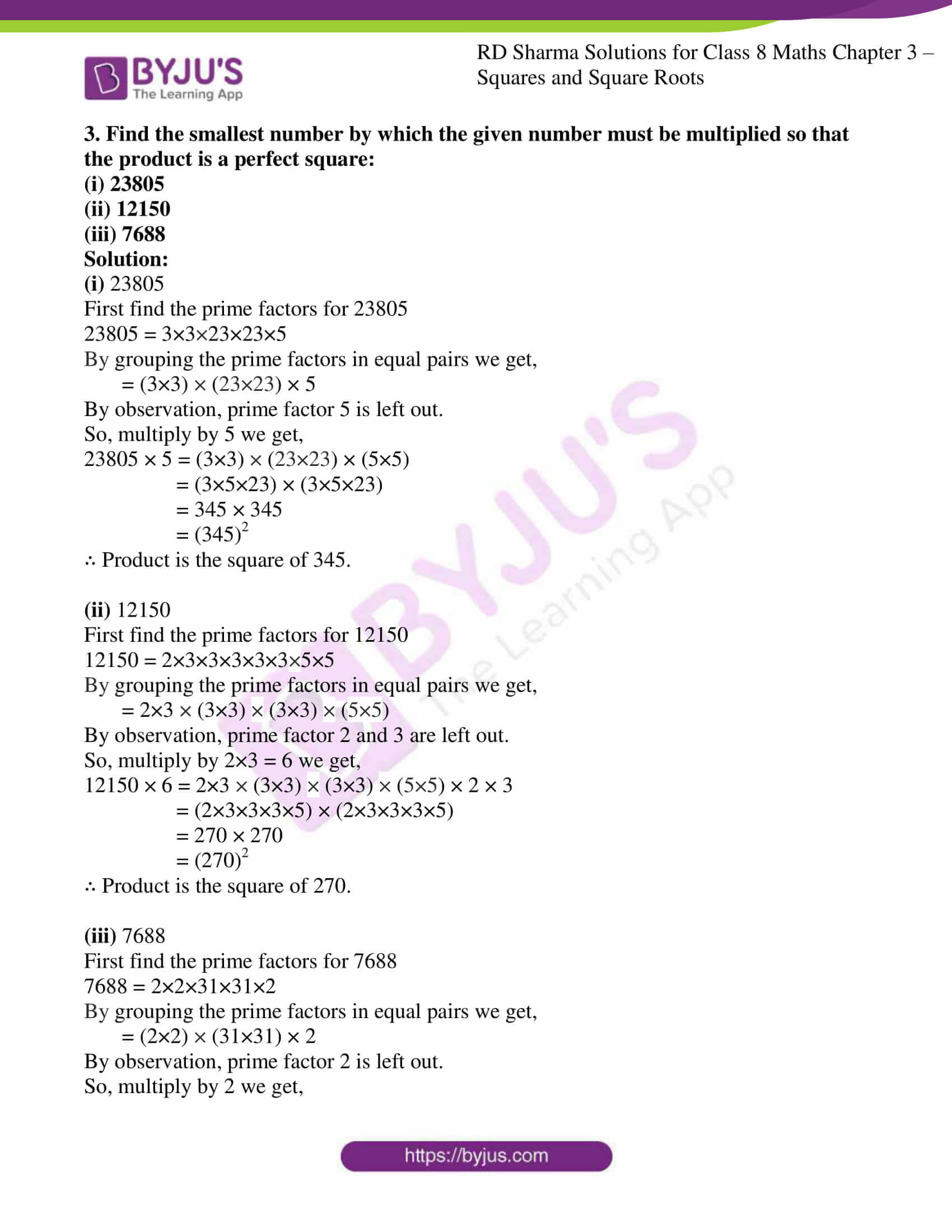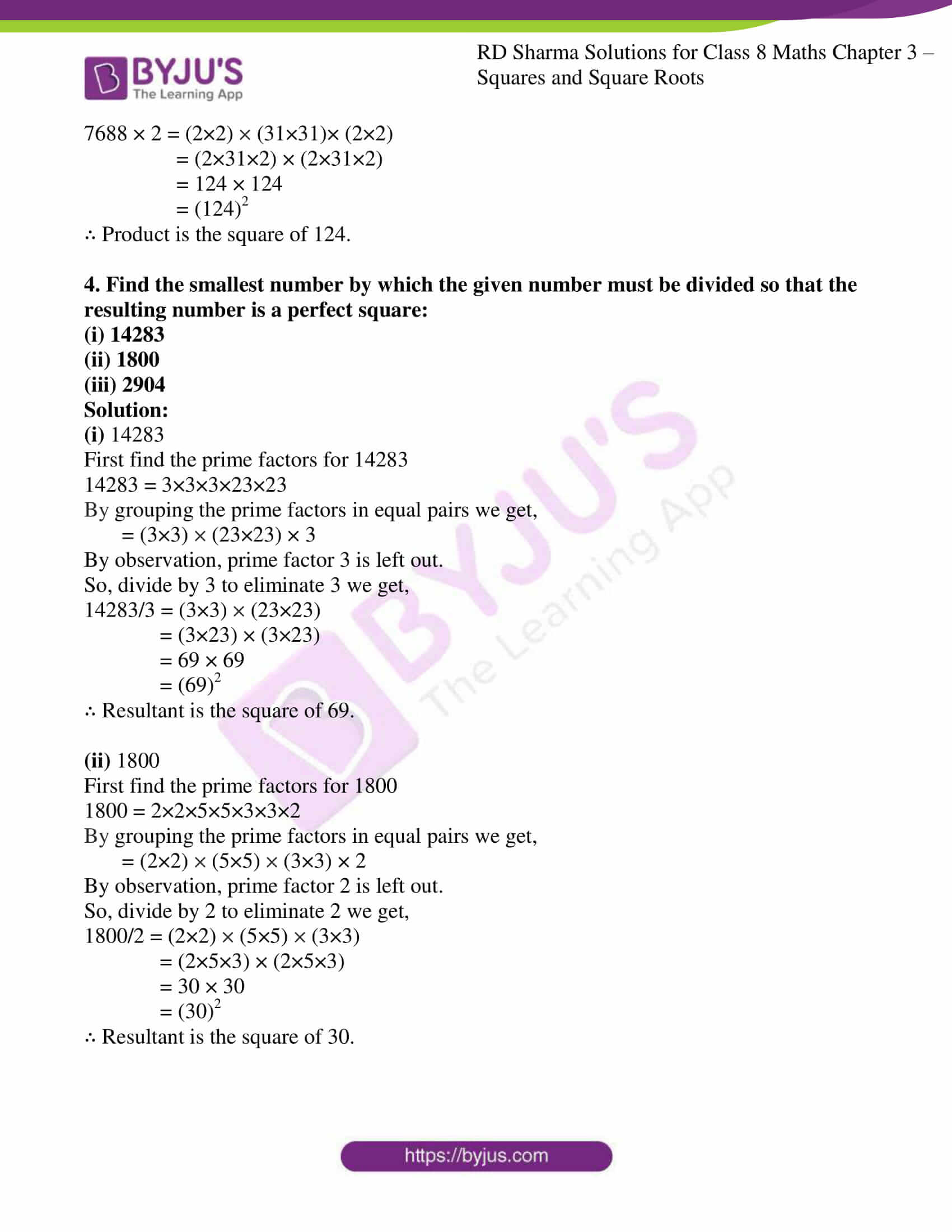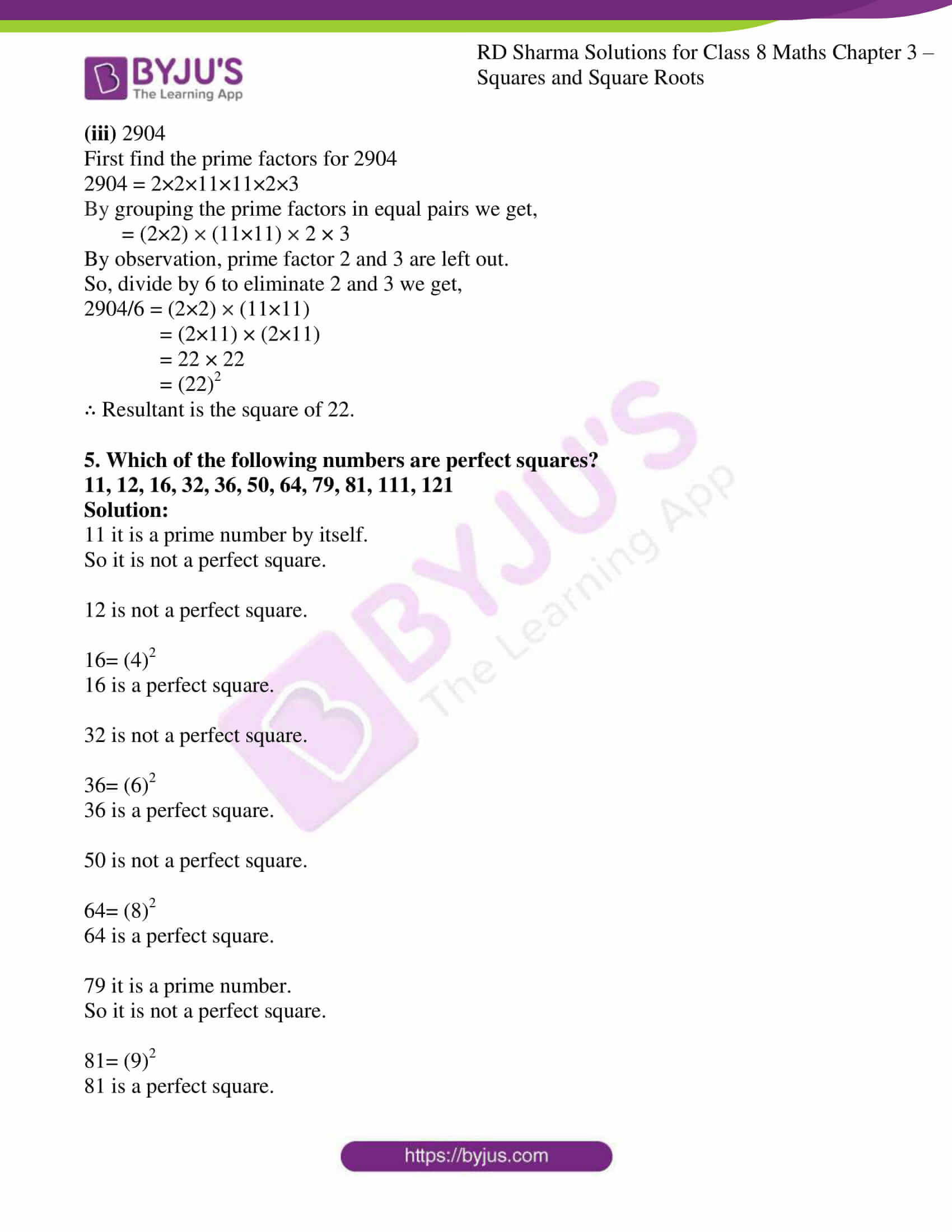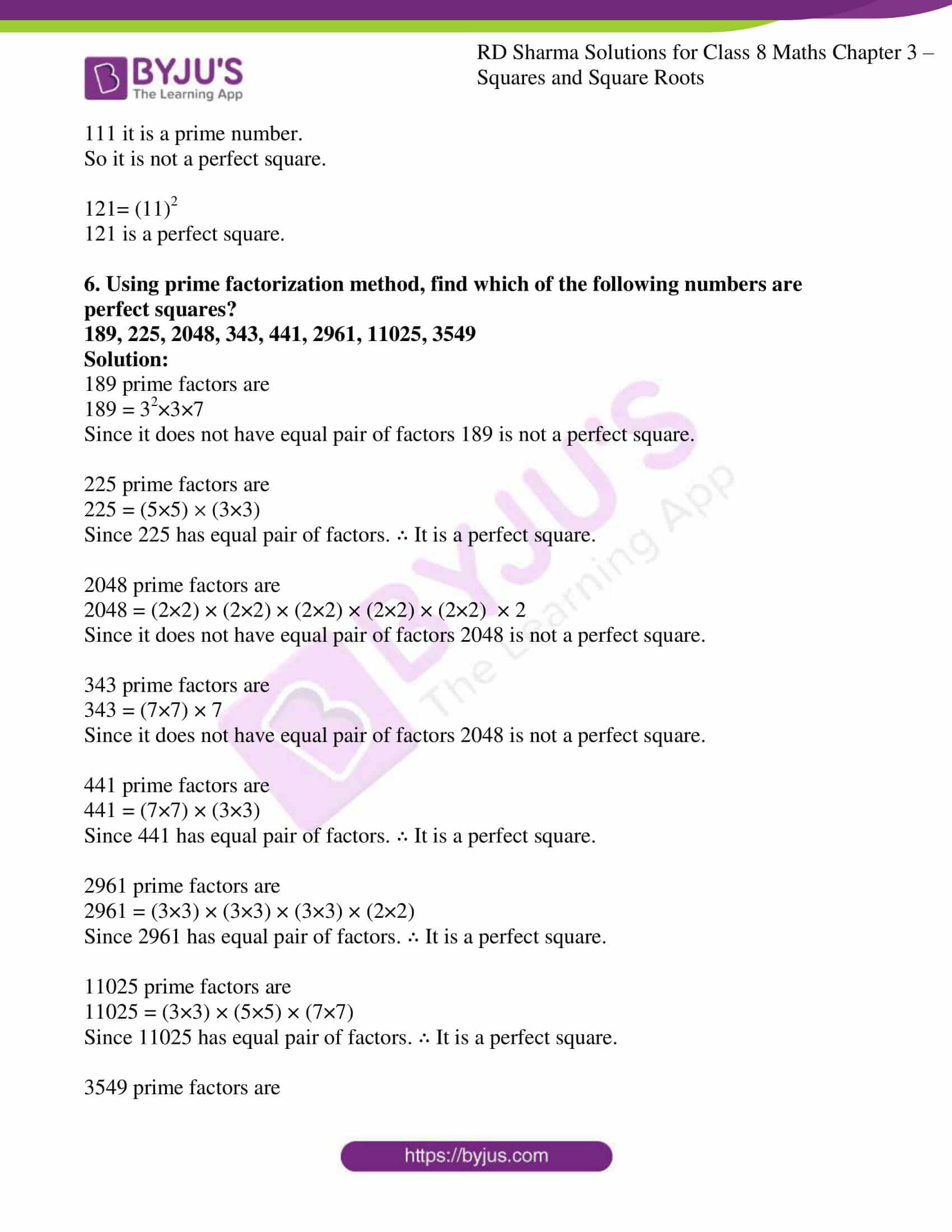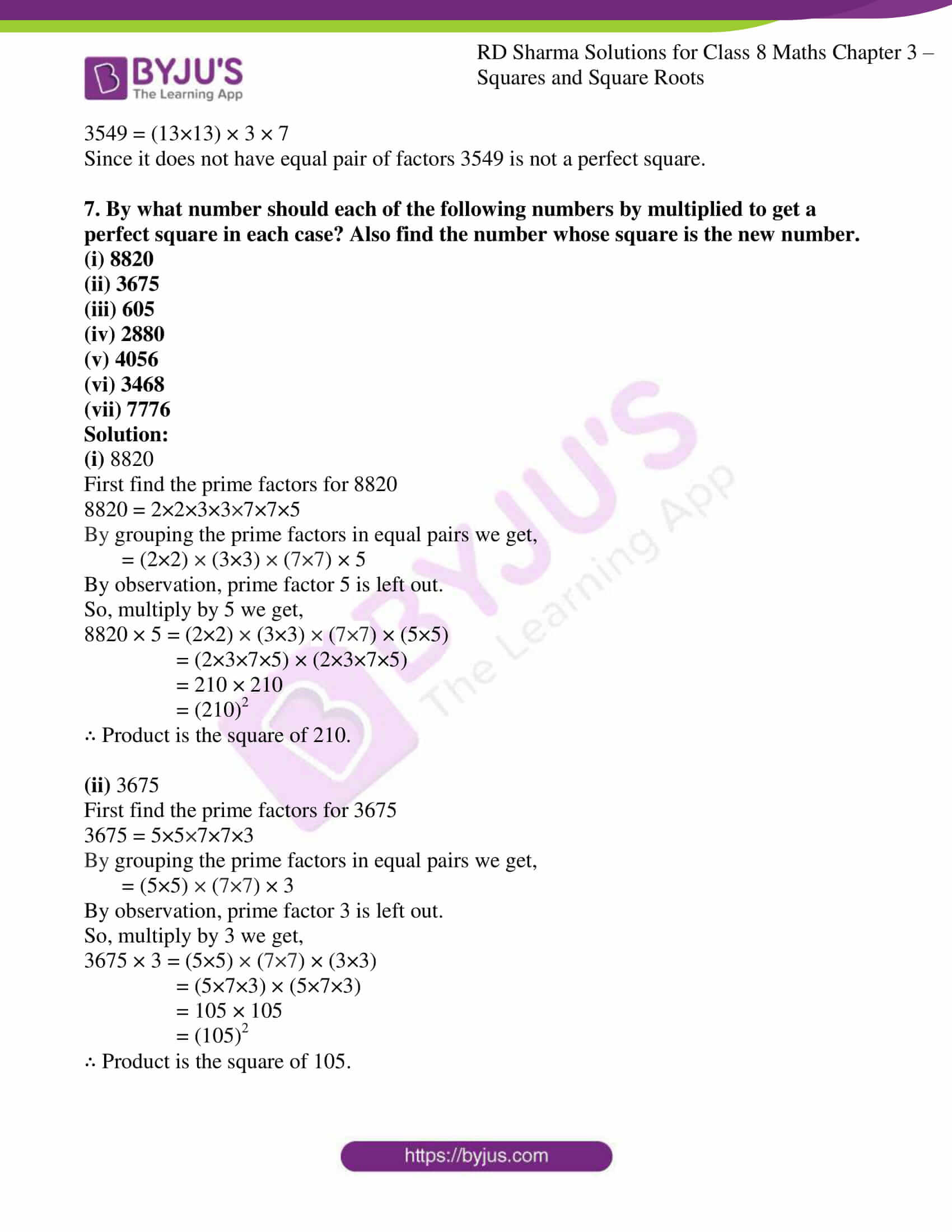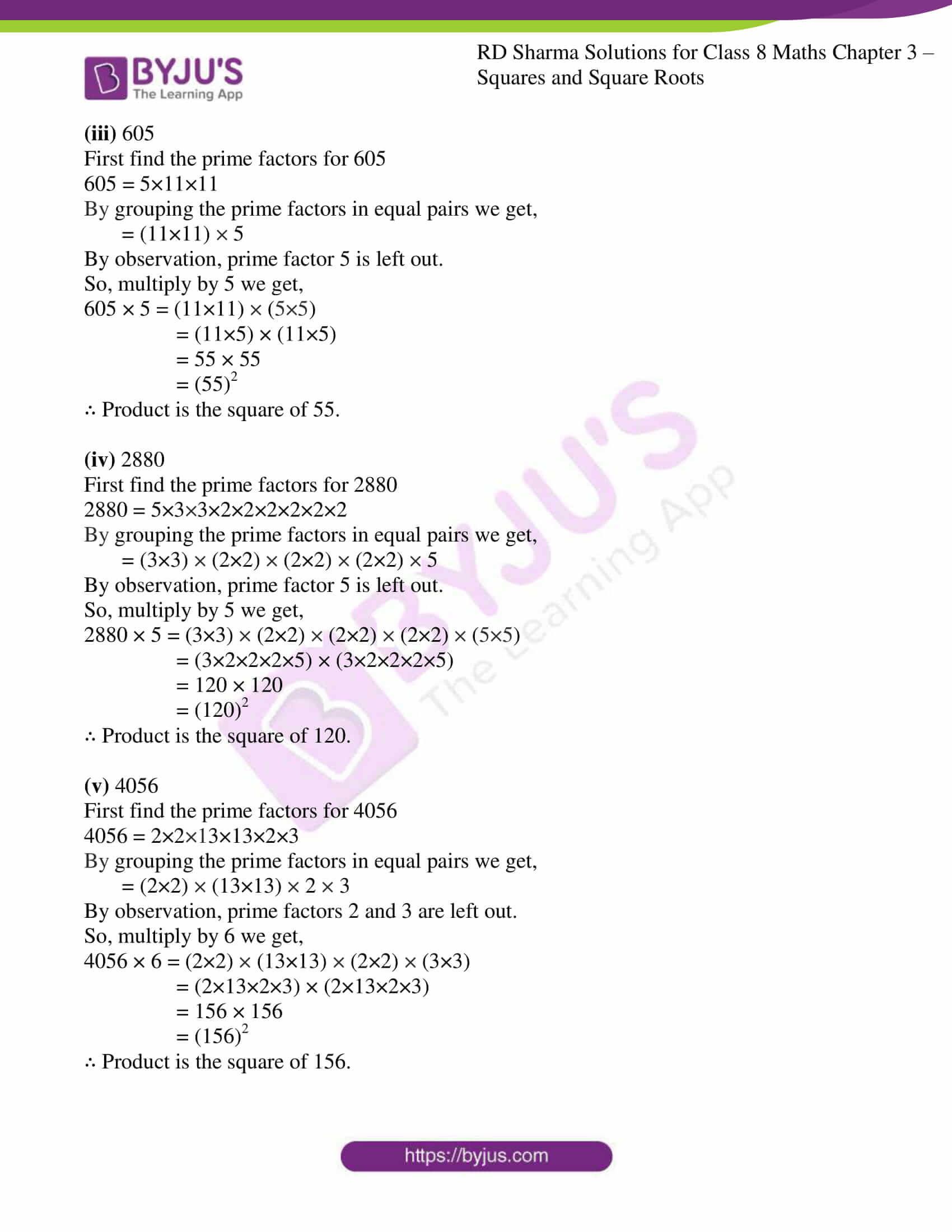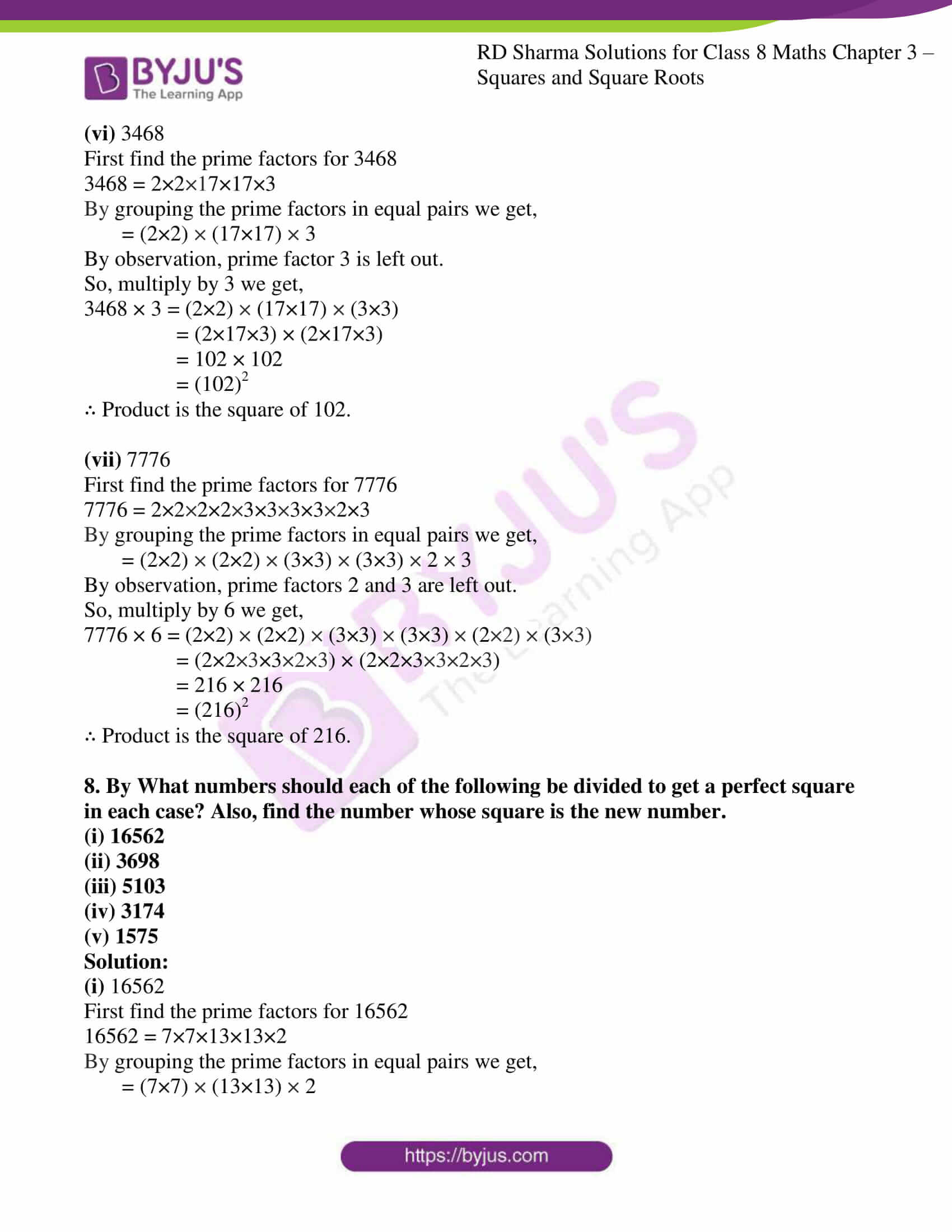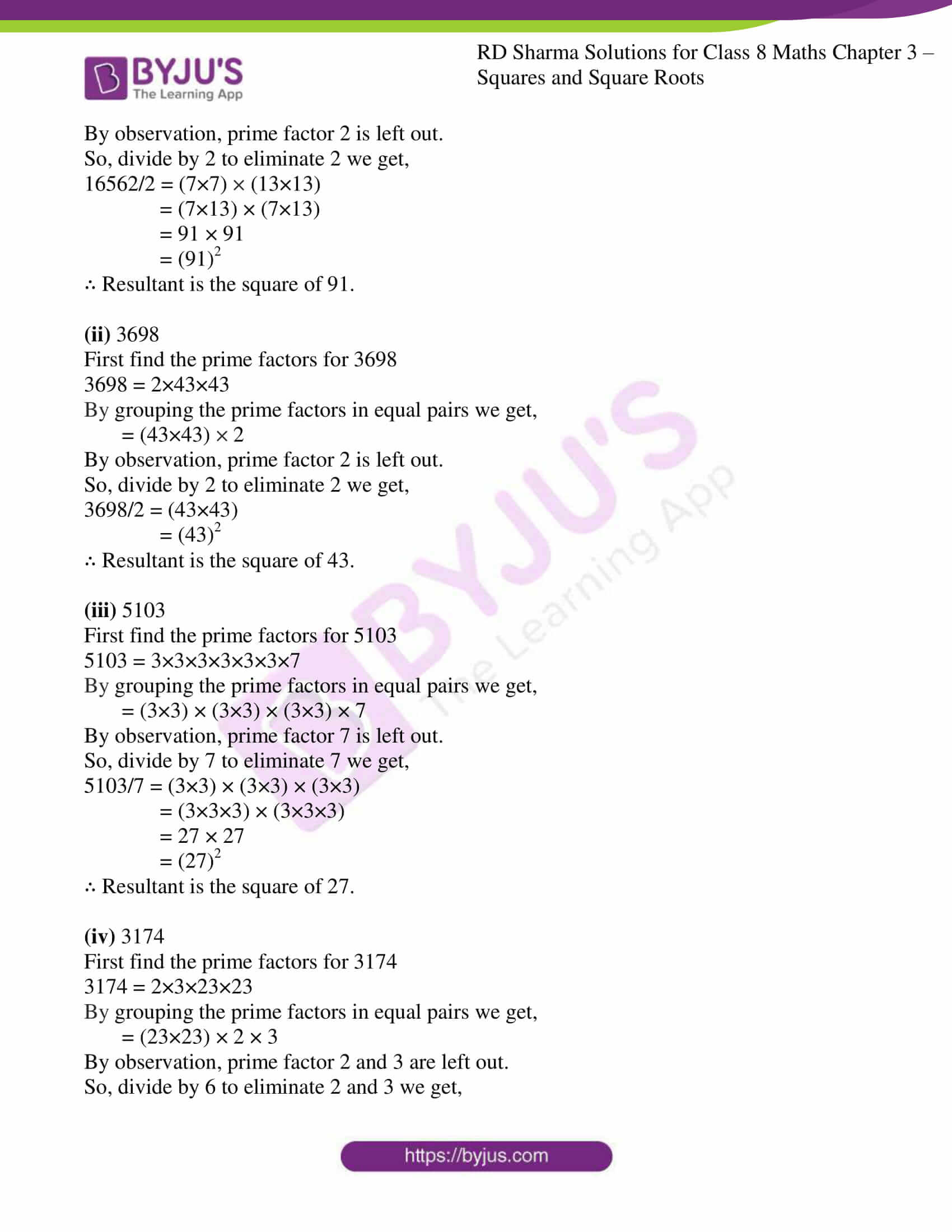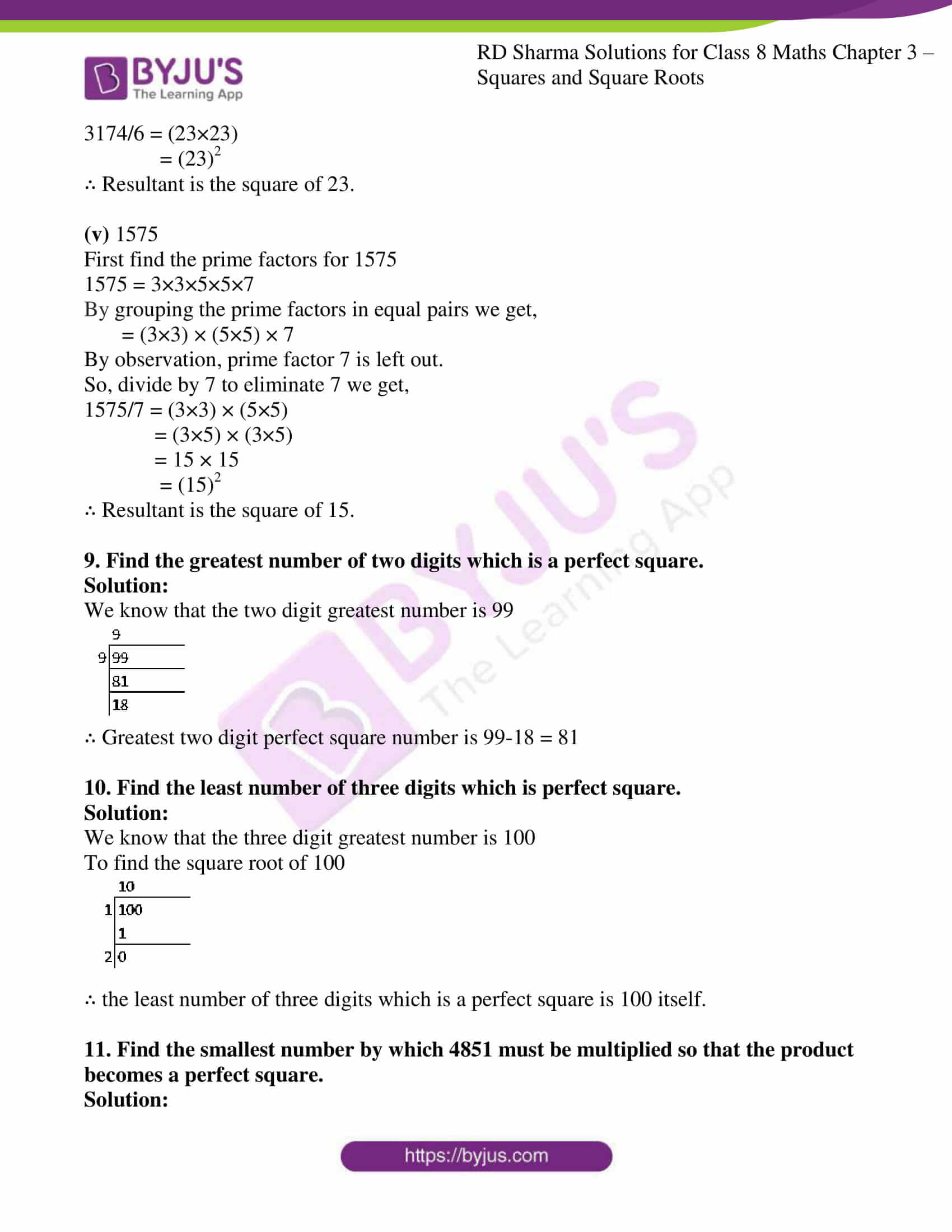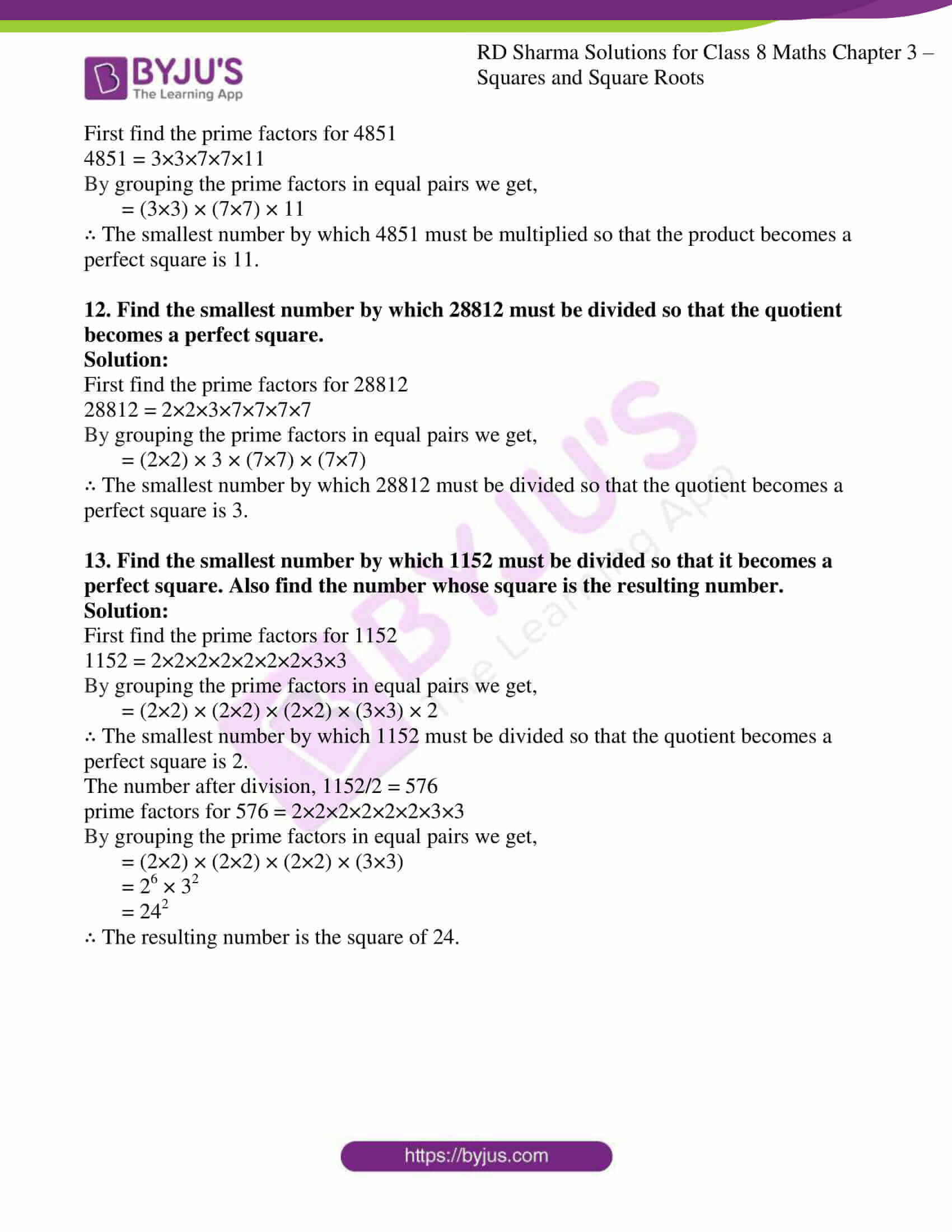### Access Answers to RD Sharma Solutions for Class 8 Maths Exercise 3.1 Chapter 3 Squares and Square Roots

#### EXERCISE 3.1 PAGE NO: 3.4

1. Which of the following numbers are perfect squares?

(i) 484

(ii) 625

(iii) 576

(iv) 941

(v) 961

(vi) 2500

Solution:

(i) 484

First find the prime factors for 484

484 = 2×2×11×11

By grouping the prime factors in equal pairs we get,

= (2×2) × (11×11)

By observation, none of the prime factors are left out.

∴ 484 is a perfect square.

(ii) 625

First find the prime factors for 625

625 = 5×5×5×5

By grouping the prime factors in equal pairs we get,

= (5×5) × (5×5)

By observation, none of the prime factors are left out.

∴ 625 is a perfect square.

(iii) 576

First find the prime factors for 576

576 = 2×2×2×2×2×2×3×3

By grouping the prime factors in equal pairs we get,

= (2×2) × (2×2) × (2×2) × (3×3)

By observation, none of the prime factors are left out.

∴ 576 is a perfect square.

(iv) 941

First find the prime factors for 941

941 = 941 × 1

We know that 941 itself is a prime factor.

∴ 941 is not a perfect square.

(v) 961

First find the prime factors for 961

961 = 31×31

By grouping the prime factors in equal pairs we get,

= (31×31)

By observation, none of the prime factors are left out.

∴ 961 is a perfect square.

(vi) 2500

First find the prime factors for 2500

2500 = 2×2×5×5×5×5

By grouping the prime factors in equal pairs we get,

= (2×2) × (5×5) × (5×5)

By observation, none of the prime factors are left out.

∴ 2500 is a perfect square.

2. Show that each of the following numbers is a perfect square. Also find the number whose square is the given number in each case:
(i) 1156
(ii) 2025
(iii) 14641
(iv) 4761

Solution:

(i) 1156

First find the prime factors for 1156

1156 = 2×2×17×17

By grouping the prime factors in equal pairs we get,

= (2×2) × (17×17)

By observation, none of the prime factors are left out.

∴ 1156 is a perfect square.

To find the square of the given number

1156 = (2×17) × (2×17)

= 34 × 34

= (34)2

∴ 1156 is a square of 34.

(ii) 2025

First find the prime factors for 2025

2025 = 3×3×3×3×5×5

By grouping the prime factors in equal pairs we get,

= (3×3) × (3×3) × (5×5)

By observation, none of the prime factors are left out.

∴ 2025 is a perfect square.

To find the square of the given number

2025 = (3×3×5) × (3×3×5)

= 45 × 45

= (45)2

∴ 2025 is a square of 45.

(iii) 14641

First find the prime factors for 14641

14641 = 11×11×11×11

By grouping the prime factors in equal pairs we get,

= (11×11) × (11×11)

By observation, none of the prime factors are left out.

∴ 14641 is a perfect square.

To find the square of the given number

14641 = (11×11) × (11×11)

= 121 × 121

= (121)2

∴ 14641 is a square of 121.

(iv) 4761

First find the prime factors for 4761

4761 = 3×3×23×23

By grouping the prime factors in equal pairs we get,

= (3×3) × (23×23)

By observation, none of the prime factors are left out.

∴ 4761 is a perfect square.

To find the square of the given number

4761 = (3×23) × (3×23)

= 69 × 69

= (69)2

∴ 4761 is a square of 69.

3. Find the smallest number by which the given number must be multiplied so that the product is a perfect square:
(i) 23805
(ii) 12150
(iii) 7688

Solution:

(i) 23805

First find the prime factors for 23805

23805 = 3×3×23×23×5

By grouping the prime factors in equal pairs we get,

= (3×3) × (23×23) × 5

By observation, prime factor 5 is left out.

So, multiply by 5 we get,

23805 × 5 = (3×3) × (23×23) × (5×5)

= (3×5×23) × (3×5×23)

= 345 × 345

= (345)2

∴ Product is the square of 345.

(ii) 12150

First find the prime factors for 12150

12150 = 2×3×3×3×3×3×5×5

By grouping the prime factors in equal pairs we get,

= 2×3 × (3×3) × (3×3) × (5×5)

By observation, prime factor 2 and 3 are left out.

So, multiply by 2×3 = 6 we get,

12150 × 6 = 2×3 × (3×3) × (3×3) × (5×5) × 2 × 3

= (2×3×3×3×5) × (2×3×3×3×5)

= 270 × 270

= (270)2

∴ Product is the square of 270.

(iii) 7688

First find the prime factors for 7688

7688 = 2×2×31×31×2

By grouping the prime factors in equal pairs we get,

= (2×2) × (31×31) × 2

By observation, prime factor 2 is left out.

So, multiply by 2 we get,

7688 × 2 = (2×2) × (31×31)× (2×2)

= (2×31×2) × (2×31×2)

= 124 × 124

= (124)2

∴ Product is the square of 124.

4. Find the smallest number by which the given number must be divided so that the resulting number is a perfect square:
(i) 14283
(ii) 1800
(iii) 2904

Solution:

(i) 14283

First find the prime factors for 14283

14283 = 3×3×3×23×23

By grouping the prime factors in equal pairs we get,

= (3×3) × (23×23) × 3

By observation, prime factor 3 is left out.

So, divide by 3 to eliminate 3 we get,

14283/3 = (3×3) × (23×23)

= (3×23) × (3×23)

= 69 × 69

= (69)2

∴ Resultant is the square of 69.

(ii) 1800

First find the prime factors for 1800

1800 = 2×2×5×5×3×3×2

By grouping the prime factors in equal pairs we get,

= (2×2) × (5×5) × (3×3) × 2

By observation, prime factor 2 is left out.

So, divide by 2 to eliminate 2 we get,

1800/2 = (2×2) × (5×5) × (3×3)

= (2×5×3) × (2×5×3)

= 30 × 30

= (30)2

∴ Resultant is the square of 30.

(iii) 2904

First find the prime factors for 2904

2904 = 2×2×11×11×2×3

By grouping the prime factors in equal pairs we get,

= (2×2) × (11×11) × 2 × 3

By observation, prime factor 2 and 3 are left out.

So, divide by 6 to eliminate 2 and 3 we get,

2904/6 = (2×2) × (11×11)

= (2×11) × (2×11)

= 22 × 22

= (22)2

∴ Resultant is the square of 22.

5. Which of the following numbers are perfect squares?
11, 12, 16, 32, 36, 50, 64, 79, 81, 111, 121

Solution:

11 it is a prime number by itself.

So it is not a perfect square.

12 is not a perfect square.

16= (4)2

16 is a perfect square.

32 is not a perfect square.

36= (6)2

36 is a perfect square.

50 is not a perfect square.

64= (8)2

64 is a perfect square.

79 it is a prime number.

So it is not a perfect square.

81= (9)2

81 is a perfect square.

111 it is a prime number.

So it is not a perfect square.

121= (11)2

121 is a perfect square.

6. Using prime factorization method, find which of the following numbers are perfect squares?
189, 225, 2048, 343, 441, 2961, 11025, 3549

Solution:

189 prime factors are

189 = 32×3×7

Since it does not have equal pair of factors 189 is not a perfect square.

225 prime factors are

225 = (5×5) × (3×3)

Since 225 has equal pair of factors. ∴ It is a perfect square.

2048 prime factors are

2048 = (2×2) × (2×2) × (2×2) × (2×2) × (2×2) × 2

Since it does not have equal pair of factors 2048 is not a perfect square.

343 prime factors are

343 = (7×7) × 7

Since it does not have equal pair of factors 2048 is not a perfect square.

441 prime factors are

441 = (7×7) × (3×3)

Since 441 has equal pair of factors. ∴ It is a perfect square.

2961 prime factors are

2961 = (3×3) × (3×3) × (3×3) × (2×2)

Since 2961 has equal pair of factors. ∴ It is a perfect square.

11025 prime factors are

11025 = (3×3) × (5×5) × (7×7)

Since 11025 has equal pair of factors. ∴ It is a perfect square.

3549 prime factors are

3549 = (13×13) × 3 × 7

Since it does not have equal pair of factors 3549 is not a perfect square.

7. By what number should each of the following numbers by multiplied to get a perfect square in each case? Also find the number whose square is the new number.

(i) 8820

(ii) 3675
(iii) 605

(iv) 2880
(v) 4056

(vi) 3468
(vii) 7776

Solution:

(i) 8820

First find the prime factors for 8820

8820 = 2×2×3×3×7×7×5

By grouping the prime factors in equal pairs we get,

= (2×2) × (3×3) × (7×7) × 5

By observation, prime factor 5 is left out.

So, multiply by 5 we get,

8820 × 5 = (2×2) × (3×3) × (7×7) × (5×5)

= (2×3×7×5) × (2×3×7×5)

= 210 × 210

= (210)2

∴ Product is the square of 210.

(ii) 3675

First find the prime factors for 3675

3675 = 5×5×7×7×3

By grouping the prime factors in equal pairs we get,

= (5×5) × (7×7) × 3

By observation, prime factor 3 is left out.

So, multiply by 3 we get,

3675 × 3 = (5×5) × (7×7) × (3×3)

= (5×7×3) × (5×7×3)

= 105 × 105

= (105)2

∴ Product is the square of 105.

(iii) 605

First find the prime factors for 605

605 = 5×11×11

By grouping the prime factors in equal pairs we get,

= (11×11) × 5

By observation, prime factor 5 is left out.

So, multiply by 5 we get,

605 × 5 = (11×11) × (5×5)

= (11×5) × (11×5)

= 55 × 55

= (55)2

∴ Product is the square of 55.

(iv) 2880

First find the prime factors for 2880

2880 = 5×3×3×2×2×2×2×2×2

By grouping the prime factors in equal pairs we get,

= (3×3) × (2×2) × (2×2) × (2×2) × 5

By observation, prime factor 5 is left out.

So, multiply by 5 we get,

2880 × 5 = (3×3) × (2×2) × (2×2) × (2×2) × (5×5)

= (3×2×2×2×5) × (3×2×2×2×5)

= 120 × 120

= (120)2

∴ Product is the square of 120.

(v) 4056

First find the prime factors for 4056

4056 = 2×2×13×13×2×3

By grouping the prime factors in equal pairs we get,

= (2×2) × (13×13) × 2 × 3

By observation, prime factors 2 and 3 are left out.

So, multiply by 6 we get,

4056 × 6 = (2×2) × (13×13) × (2×2) × (3×3)

= (2×13×2×3) × (2×13×2×3)

= 156 × 156

= (156)2

∴ Product is the square of 156.

(vi) 3468

First find the prime factors for 3468

3468 = 2×2×17×17×3

By grouping the prime factors in equal pairs we get,

= (2×2) × (17×17) × 3

By observation, prime factor 3 is left out.

So, multiply by 3 we get,

3468 × 3 = (2×2) × (17×17) × (3×3)

= (2×17×3) × (2×17×3)

= 102 × 102

= (102)2

∴ Product is the square of 102.

(vii) 7776

First find the prime factors for 7776

7776 = 2×2×2×2×3×3×3×3×2×3

By grouping the prime factors in equal pairs we get,

= (2×2) × (2×2) × (3×3) × (3×3) × 2 × 3

By observation, prime factors 2 and 3 are left out.

So, multiply by 6 we get,

7776 × 6 = (2×2) × (2×2) × (3×3) × (3×3) × (2×2) × (3×3)

= (2×2×3×3×2×3) × (2×2×3×3×2×3)

= 216 × 216

= (216)2

∴ Product is the square of 216.

8. By What numbers should each of the following be divided to get a perfect square in each case? Also, find the number whose square is the new number.
(i) 16562
(ii) 3698
(iii) 5103
(iv) 3174
(v) 1575

Solution:

(i) 16562

First find the prime factors for 16562

16562 = 7×7×13×13×2

By grouping the prime factors in equal pairs we get,

= (7×7) × (13×13) × 2

By observation, prime factor 2 is left out.

So, divide by 2 to eliminate 2 we get,

16562/2 = (7×7) × (13×13)

= (7×13) × (7×13)

= 91 × 91

= (91)2

∴ Resultant is the square of 91.

(ii) 3698

First find the prime factors for 3698

3698 = 2×43×43

By grouping the prime factors in equal pairs we get,

= (43×43) × 2

By observation, prime factor 2 is left out.

So, divide by 2 to eliminate 2 we get,

3698/2 = (43×43)

= (43)2

∴ Resultant is the square of 43.

(iii) 5103

First find the prime factors for 5103

5103 = 3×3×3×3×3×3×7

By grouping the prime factors in equal pairs we get,

= (3×3) × (3×3) × (3×3) × 7

By observation, prime factor 7 is left out.

So, divide by 7 to eliminate 7 we get,

5103/7 = (3×3) × (3×3) × (3×3)

= (3×3×3) × (3×3×3)

= 27 × 27

= (27)2

∴ Resultant is the square of 27.

(iv) 3174

First find the prime factors for 3174

3174 = 2×3×23×23

By grouping the prime factors in equal pairs we get,

= (23×23) × 2 × 3

By observation, prime factor 2 and 3 are left out.

So, divide by 6 to eliminate 2 and 3 we get,

3174/6 = (23×23)

= (23)2

∴ Resultant is the square of 23.

(v) 1575

First find the prime factors for 1575

1575 = 3×3×5×5×7

By grouping the prime factors in equal pairs we get,

= (3×3) × (5×5) × 7

By observation, prime factor 7 is left out.

So, divide by 7 to eliminate 7 we get,

1575/7 = (3×3) × (5×5)

= (3×5) × (3×5)

= 15 × 15

= (15)2

∴ Resultant is the square of 15.

9. Find the greatest number of two digits which is a perfect square.

Solution:

We know that the two digit greatest number is 99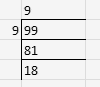∴ Greatest two digit perfect square number is 99-18 = 81

10. Find the least number of three digits which is perfect square.

Solution:

We know that the three digit greatest number is 100

To find the square root of 100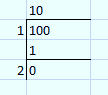∴ the least number of three digits which is a perfect square is 100 itself.

11. Find the smallest number by which 4851 must be multiplied so that the product becomes a perfect square.

Solution:

First find the prime factors for 4851

4851 = 3×3×7×7×11

By grouping the prime factors in equal pairs we get,

= (3×3) × (7×7) × 11

∴ The smallest number by which 4851 must be multiplied so that the product becomes a perfect square is 11.

12. Find the smallest number by which 28812 must be divided so that the quotient becomes a perfect square.

Solution:

First find the prime factors for 28812

28812 = 2×2×3×7×7×7×7

By grouping the prime factors in equal pairs we get,

= (2×2) × 3 × (7×7) × (7×7)

∴ The smallest number by which 28812 must be divided so that the quotient becomes a perfect square is 3.

13. Find the smallest number by which 1152 must be divided so that it becomes a perfect square. Also find the number whose square is the resulting number.

Solution:

First find the prime factors for 1152

1152 = 2×2×2×2×2×2×2×3×3

By grouping the prime factors in equal pairs we get,

= (2×2) × (2×2) × (2×2) × (3×3) × 2

∴ The smallest number by which 1152 must be divided so that the quotient becomes a perfect square is 2.

The number after division, 1152/2 = 576

prime factors for 576 = 2×2×2×2×2×2×3×3

By grouping the prime factors in equal pairs we get,

= (2×2) × (2×2) × (2×2) × (3×3)

= 26 × 32

= 242

∴ The resulting number is the square of 24.

## RD Sharma Solutions for Class 8 Maths Exercise 3.1 Chapter 3 – Squares and Square Roots

Exercise 3.1 of RD Sharma Solutions for Chapter 3 Squares and Square Roots, deals with the basic concepts related to a perfect square as well as the fundamental explanations of properties of perfect squares.

The RD Sharma Solutions can help the students in practising and learning each and every concept as it provides solutions to all questions asked in the RD Sharma textbook. Those who aim to score high in the Maths of Class 8 are advised to practice all the questions present in RD Sharma as many times as possible.